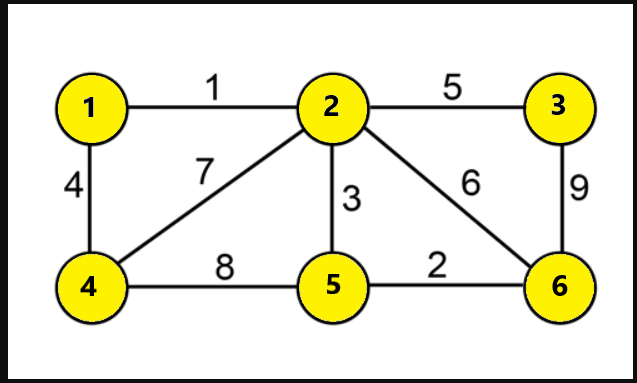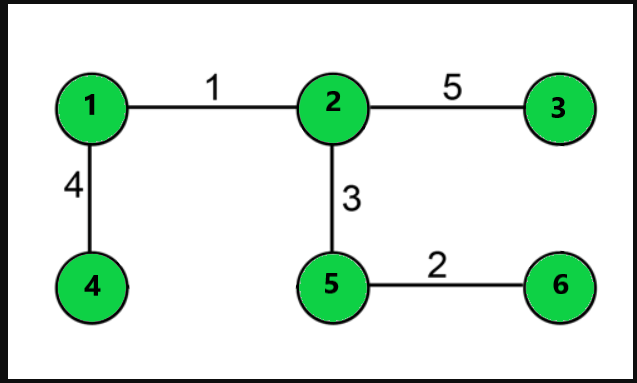# Boruvka’s Algorithm with Implementation in Java

In this article, we will have a look at another interesting algorithm related to Graph Theory – Boruvka’s Algorithm. We will also look at a problem with respect to this algorithm, discuss our approach and analyze the complexities.

Boruvka’s Algorithm is mainly used to find or derive a Minimum Spanning Tree of an Edge-weighted Graph. Let us have a quick look at the concept of a Minimum Spanning Tree. A Minimum Spanning Tree or a MST is a subset of edges of a Weighted, Un-directed graph; such that it connects all the vertices together. The resultant subset of the graph must have no cycles or loops within it. Moreover, It should have minimum possible total weight of the edges connecting the tree.

Note: The Minimum Spanning Tree should connect all its vertices. A disconnected graph is not a MST.

Let us understand this with an example, consider this graph:The above shown graph is an Edge-Weighted, undirected graph with 6 vertices. The minimum Spanning tree of the above graph looks like this:### Explanation:

The above image shows the Minimum Spanning tree of Graph G, as it connects all the vertices together. The resultant graph has no loops or cycles within it. After trying out various examples we select the smallest edge from each vertex and connect the two vertices together. We avoid connecting those edges which are already processed as it may form a cycle. The Minimum Possible Weight obtained from this MST adding all the weights from respective edges: 1 ( Edge 1 to 2 ) + 4 ( Edge 1 to 4 ) + 5 ( Edge 2 to 3 ) + 3 ( Edge 2 to 5 ) +2  (Edge 5 to 6 ); so Total Weight of MST = 15.

Note: The Maximum Number of Edges present in a Minimum Spanning Tree = Number of Vertices – 1.

## Boruvka’s Algorithm

Now let us see how Boruvka’s Algorithm is helpful in finding the MST of a graph.

• The idea is to separate all nodes at first, then process each node one by one by connecting nodes together from different components.
• For each node, we find the edge with least weight and connect them to form a component. Then we jump to the next vertex.
• After this, for each component, we choose the smallest or cheapest edge so that we get disconnected components of graphs. Then we combine the graph using the above process. If any loop or cycle found we ignore those edges.
• After getting all the disconnected components we try connecting them following the above steps. Each repetition of this process reduces the number of nodes, within each connected component of the graph, to at most half of this former value, so after logarithmically many repetitions the process finishes.
• At the end, the weight of edges we add from the Minimum Spanning Tree.

### Implementation in Java

Step 1:

We represent the Graph using a class with three fields: V, U, and Cost. V is the source vertex , U is the destination and Cost is the weight between V and U. We use two arrays Parent and Min. The Parent Array stores the parent of node and Min stores the Minimum outgoing edge for each pair (v,u). For ith node we initialize its parent value to the same node.

Step 2:

At first we set the number of Components to the number of vertices n. For each component we initialize Min as -1, indicating there is no cheapest edge. For each node in our graph if its source and end vertex are part of same component we do not process them. Otherwise, for each vertex we take their root or parent node and check if it is minimum weighted edge.

Step 3:

Then, we iterate through each component, if there is an each edge for pair (u,v) we merge them into a single component. Before merging we check if the nodes are from same component, on doing this we avoid merging two nodes into same component which might create a loop or cycle. If we are able to merge the two components we add their edge weight. We repeat these steps for each component. This makes sure all edges are visited at least once and on each iteration, we skip (log n) number of nodes.

Now let us look at the implementation of the above in Java code:

Output:

Note: We take Graph array of size 10 for total no. of edges are 9 as discussed in the example above and vertices are named from 1. The same is for Parent and Min array, we take size 7 for 6 vertices.

We have implemented the code for the same example as shown above. Now let us have a quick look at the complexities.

Time Complexity: For N nodes of graph, we have E edges to check the minimum weighted edge we have to iterate through all the edges and on each iteration the total nodes to be processed decreases logarithmically. So the overall complexity is O( E * log(N) ) .

Space Complexity: We require extra space to store the Parent and Min edge with respect to each node in our graph of size equal to the total number of vertices N. So the overall complexity is O(N).

### Limitation Of Boruvka’s Algorithm

We can see in the above example that we used the graph with edges having distinct weight. This is a limitation for this algorithm which requires the graph to be Edge-Weighted but with Distinct Weights. If edges do not have distinct weights, then a consistent tie-breaking rule can be used. An optimization is to remove each edge in Graph G that is found to connect two vertices in the same component as each other.

So that’s it for the article you can try out this algorithm and dry run with various examples to have a clear idea. You can also execute this code to have a better understanding.

Feel free to leave your suggestions/doubts in the comment section below.